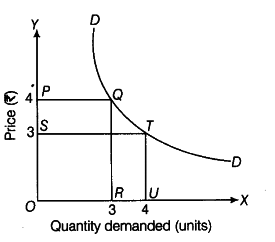# When does a demand curve take the shape of a rectangular hyperbola?

If the equation of a demand curve takes the form pq = e, where e is constant, irrespective of the values of p and q, then the demand curve that we get is a rectangular hyper¬bola, implying that on each and every point on the curve,
the area underlying is the same. In the graph depicted above, point Q on the demand curve corresponds to area enclosed by rectangle OPQR which is 12 units [OP x OR = 4 x 3]
Also, point T on the demand curve corresponds to area enclosed by rectangle OSTU which is again 12 units [OSxOU = 3x4],
Therefore, demand curve DD is a rectangular hyperbola.